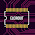# Power Amplifier Micro Driver

This time I will share about the power micro driver or super mini power with great power for the driver. This power circuit is capable of up to 75V DC symmetrical, Where this micro power amplifier can produce incredible power up to 1000W if properly coupled and using a good power supply is minimal to big power out, the current intended for this power is 20Ampere, although the power amplifier is quite simple with a less complex circuit schemes, which you can see in the picture below and see also the PCB Layout Design:

Power Amplifier Micro Driver Circuit Diagram
Component List:
R1= 100K
R2,R13= 2K2
R3, R5, R10= 100R
R4, R8, R9= 10K
R6= 470R
R7= 33K
R11, R12= 220R
C1= 100N
C2= 100uF/100V
Q1= C945
Q2= C1815
Q3, Q7= MJE340
Q4= A1837
Q5, Q6= TIP2955
Q8= C4793
Q9= TIP3055
J1= Audio Input
J2= Audio Output/ Emitter Final Transistor
J3= VCC (-) 45V-75V DC
J4= B (-) FT
J5= B (+) FT
J6= VCC (+) 45V-75V DC
GND/CT
Prototype Amplifier VIDEO

The circuit above is the only driver circuit so you have to add the final transistor as 2sc5200 2sa1943 10set much or more to add more power output. Or see final transistor power amplifier list.

Here for PCB layout that can be used as I design and later made to the size of 85mm x 62,5mm.
PCB LAYOUT DESIGN POWER AMPLIFIER MICRO DRIVER
8.5cm x 6.25cm

## 5 comments for "Power Amplifier Micro Driver "

1.This comment has been removed by the author.

2.Olá tudo bem? Esse circuito já foi testado?

3.gracias,,, muy buen proyecto,, lo necesitaba,,,Dios les bendiga mucho,,paz

4.Sir gud day.. Can i use 5 sets of c2922 / a1216? For this driver?

5.yes you can